# How to define the type of combination?

Since SCIA Engineer 18.0 it is possible to have automatic generated code combinations in your project. However, if you want to define your own combinations, it is important to know the three main types that are available:

• Eurocode
• Envelope
• Linear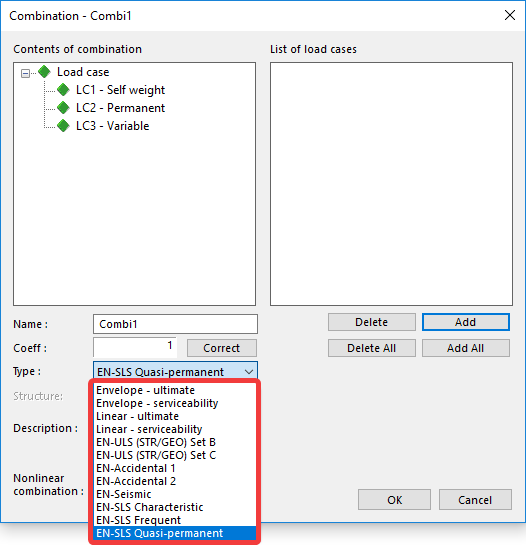The differences between these three types are the use of coefficients (load combination factors) and the defined relations between the load cases of which the combination will consist. First, the differences will be discussed. Second, the theory is put into practice by the use of an example.

# Use of coefficients

For the use of the coefficients, the following rule applies: If the type of combination is Eurocode, SCIA Engineer will automatically use the load combination factors set by the chosen National Annex of the Eurocode. For the Standard EN, this is shown in figure below.

If the type of combination is Envelope or Linear, SCIA will not use these load combination factors, but the ones inputted in the combination: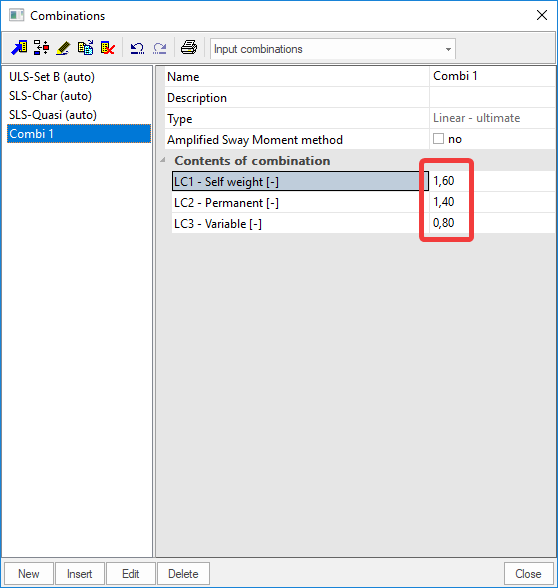# Use of relations

The relation of a load group defines how the load cases in this group are related. For a permanent load case, there is no need to define a relation since these load cases are permanently present. For a variable load case, the relation can be set in the load groups. There are three relations possible:

• Standard
• Exclusive
• Together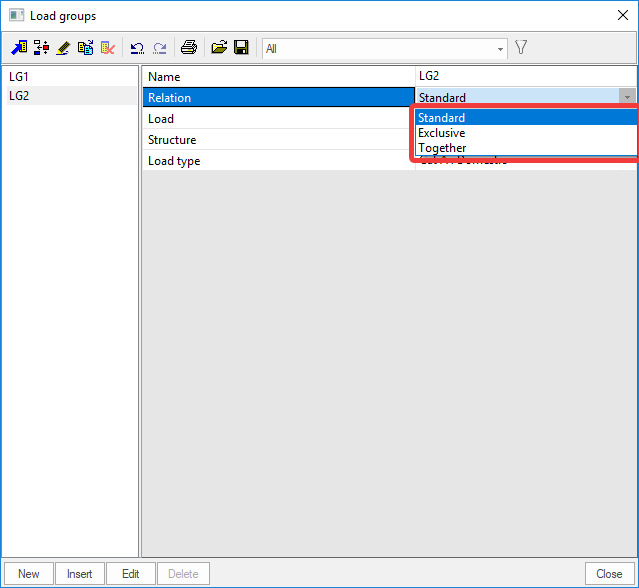Imagine the two variable load cases A and B are put into the same load group. This means for each defined relation: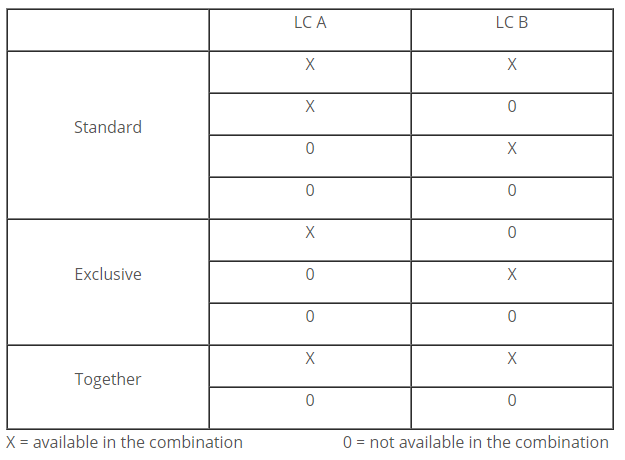# Differences in type of combination

The previous described differences are now used as follows in the three types of combination: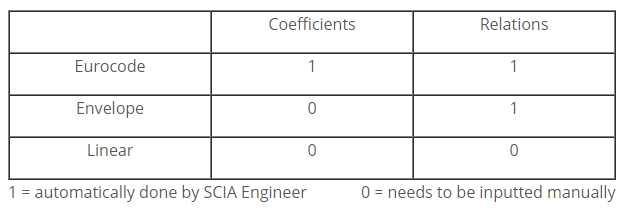Since for the Linear type you will need to input everything manually, this means that it consists of only 1 combination. For the Envelope type you will need to input the coefficients, but the defined relations are taken into account by SCIA Engineer. This means that the one Envelope combination can consist of multiple linear combinations where the relations are taken into account. The Eurocode combinations will take the coefficients from the set National Annex and the relations from the set load groups. Like the Envelope combination, this implies that one Eurocode combination can consist of multiple linear combinations where the set coefficients and relations are taken into account.

# Example

Suppose that we input the following load cases:

• LC 1: Self weight / permanent / coefficient = 1,35 / Load group 1 = permanent
• LC 2: Permanent / permanent / coefficient = 1,4 / Load group 1 = permanent
• LC 3: Variable 1 / variable / coefficient = 1,2 / Load group 2 = variable (Standard)
• LC 4: Variable 2 / variable / coefficient = 0,8 / Load group 3 = variable (Exclusive)
• LC 5: Variable 3 / variable / coefficient = 0,9 / Load group 3 = variable (Exclusive)

## Linear combination

For the Linear combination this results into only 1 combination: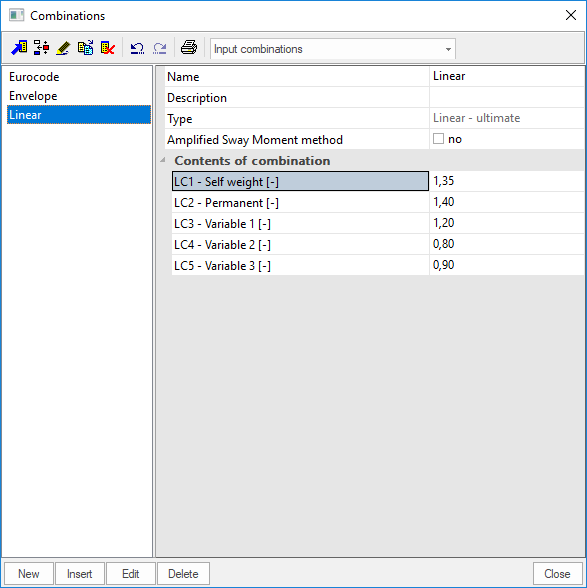This is actually a wrong result since we want LC 4 and LC 5 to be exclusive, i.e. not together in the same combination. However, the linear type will not account for the set relations in the load group!

## Envelope combination

For the Envelope combination this results into 6 linear combinations: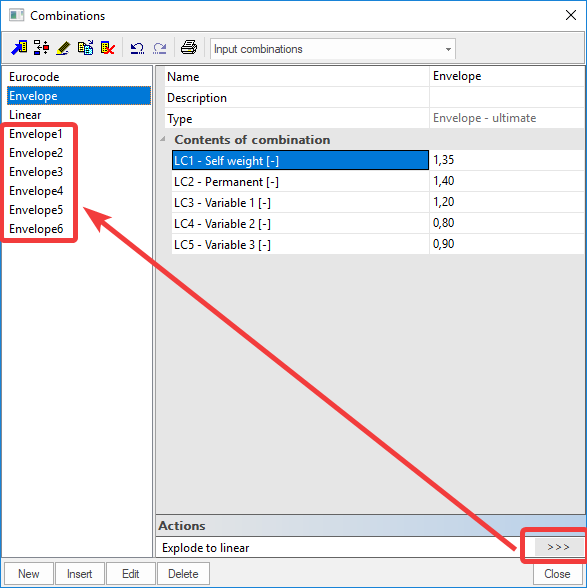The envelope combination consists of multiple linear combination because of the different relation between the load cases. Note that the coefficients remain unchanged throughout the 6 linear combinations.

Some of the 6 combinations are:

• 1,35*LC1 + 1,4*LC2
• 1,35*LC1 + 1,4*LC2 + 1,2*LC3
• 1,35*LC1 + 1,4*LC2 + 1,2*LC3 + 0,8*LC4

## Eurocode combination

For the Eurocode combination this results into 22 linear combinations: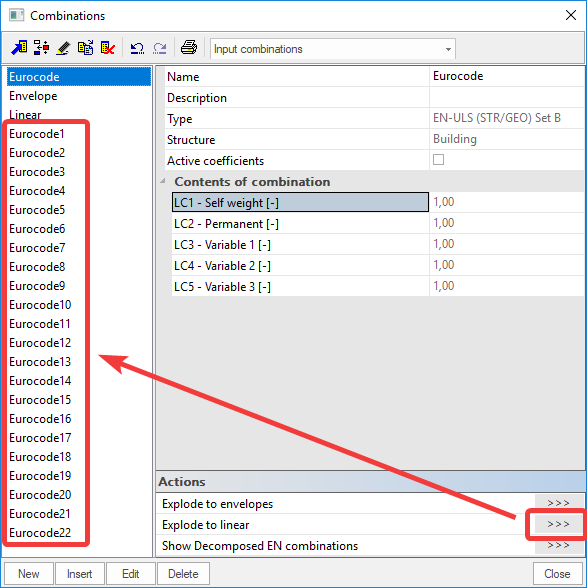The Eurocode combination consists of more linear combinations compared to the Envelope combination. As seen on the figure above, all coefficients are 1,00 and grayed out. This shows that the coefficients are inputted automatically by SCIA Engineer.

Some of the 22 linear combinations are:

• 1,35*LC1 + 1,35*LC2
• 1,00*LC1 + 1,00*LC2
• 1,35*LC1 + 1,35*LC2 + 1,5*LC3